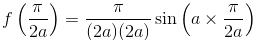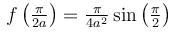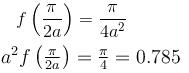Courses

# Differential Equation NAT Level - 1

## 10 Questions MCQ Test Topic wise Tests for IIT JAM Physics | Differential Equation NAT Level - 1

Description
This mock test of Differential Equation NAT Level - 1 for IIT JAM helps you for every IIT JAM entrance exam. This contains 10 Multiple Choice Questions for IIT JAM Differential Equation NAT Level - 1 (mcq) to study with solutions a complete question bank. The solved questions answers in this Differential Equation NAT Level - 1 quiz give you a good mix of easy questions and tough questions. IIT JAM students definitely take this Differential Equation NAT Level - 1 exercise for a better result in the exam. You can find other Differential Equation NAT Level - 1 extra questions, long questions & short questions for IIT JAM on EduRev as well by searching above.
*Answer can only contain numeric values
QUESTION: 1

### If the Particular Integral (P.I.) of the differential equation (D3 + 1)y = cos2x. is given by y = 1/65 (cos 2x - β sin 2x). Find the value of β

Solution: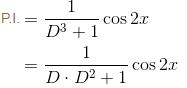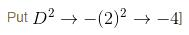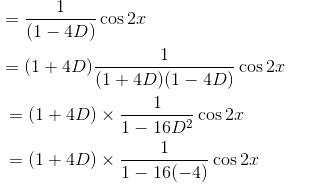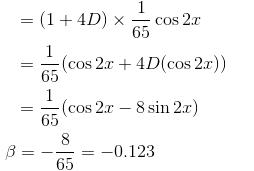*Answer can only contain numeric values
QUESTION: 2

### I.F of differential equation (y2 + 2x2y)dx + (2x3 – xy)dy = 0 is of the form xαyβ. Then find value of α + β

Solution: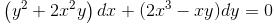Now, suppose, xh yk is IF then,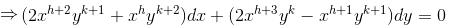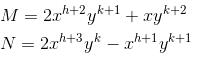At this is exact so,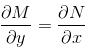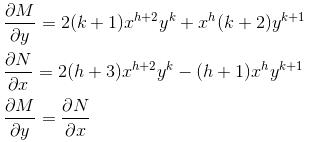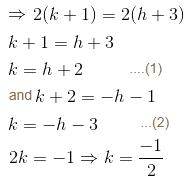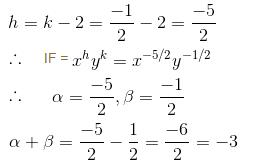*Answer can only contain numeric values
QUESTION: 3

### The IF for the Differential Equation (1 + xy)y + x (1 - xy)dy/dx = 0 is xαyβ. Then find the value of the α + β.

Solution: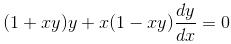Comparing this equation with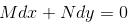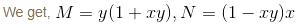Since, it is a homogeneous differential equation of form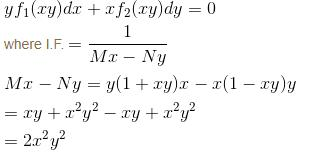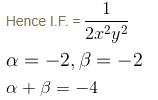*Answer can only contain numeric values
QUESTION: 4

Find the value of f(0) where f(x) is the Particular Integral (P.I.) of the Differential equation (D2 –2D + 1)y = x sin x.

Solution: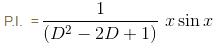Applying the formula, we get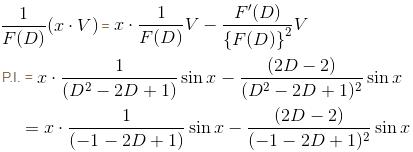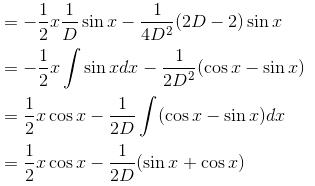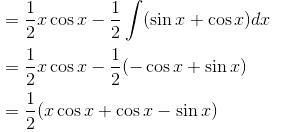∴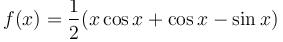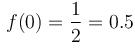*Answer can only contain numeric values
QUESTION: 5

Solve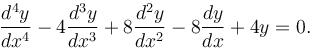In the solution find the value of constant which is in multiplication with cos x only given y(0) = 0.

Solution: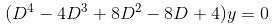Auxiliary Equation is
m– 4m3 + 8m2 – 8m + 4 = 0
(m2 – 2m + 2)2 = 0
∴  m = 1 ± i, 1 ± i
Hence, sol of equation is

y =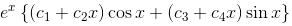Now,    y = 0 at x = 0
⇒  c1 = 0
Required constant c1 = 0.

*Answer can only contain numeric values
QUESTION: 6

Let y be a function of x satisfying dy/dx = 2x3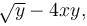y(0) = 0, then find the value of e2y(1).

Solution: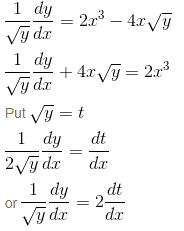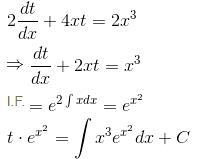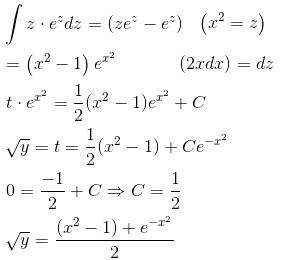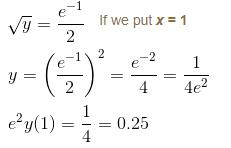*Answer can only contain numeric values
QUESTION: 7

Given a differential equation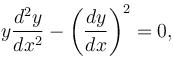y(0) = 1, y(1) = e4, then y(4) = eα . Find the value of α.

Solution: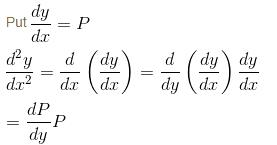∴  Equation becomes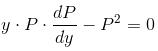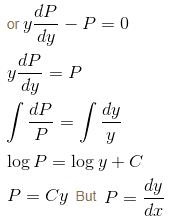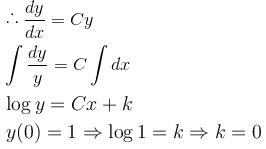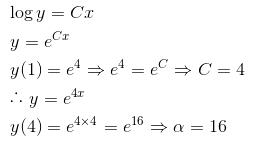*Answer can only contain numeric values
QUESTION: 8

The Particular Integral (PI) of the differential equation (D2 – 5D + 6)y = x is given as y = mx + c. Find the value of c.

Solution: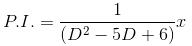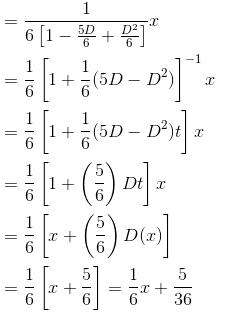y = mx + c
⇒ c = 5/36 = 0.138

*Answer can only contain numeric values
QUESTION: 9

If the differential equation (3a2y2x2 + bycosx)dx + (2sinx – 4ayx3)dy = 0 is exact then what is the value of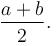a, b ≠ 0.

Solution: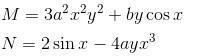For Differential equation to be exact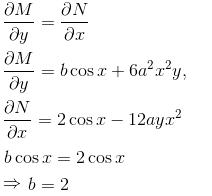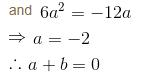*Answer can only contain numeric values
QUESTION: 10

If the Particular Integral (PI) of the Differential equation (D2 + a2)y = cos ax is given by f(x). Then find the value of a2f (π/2a).

Solution:

As, we known, the P.I. of (D2 + a2)y = cos ax is given as  x/2a sin ax
∴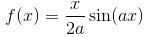Now,